# Build Your Own Support Vector Machine

Support vector machines are the canonical example of the close ties between convex optimization and machine learning. Trained on a set of labeled data (i.e. this is a supervised learning algorithm) they are algorithmically simple and can scale well to large numbers of features or data samples, and have been shown to be effective on a variety of problems. Whether classifying data (spam or not-spam) or creating regression models (by dividing the space into a large set of categories), a support vector machine is one of the go-to tools for data classification.

In this tutorial, we’ll go through the steps for building a support vector machine from scratch, in order to see what’s “under the hood” of this classic algorithm. This follows the structure in Stephen Boyd’s excellent Convex Optimization book, section 8.6- with additional content drawn from Calafiore & El Ghaoui’s Optimization Models (or on Amazon here).

# Linear Classifiers¶

The core idea of a support vector machine is to find a line -or in higher dimensions, a hyperplane- which separates two labeled classes in our training dataset. We will find a way to define this hyperplane to maximize the distance between the two datasets, and then define the hyperplane by the boundary points from the classes which touch it- the support vectors (“support points” might be an easier way to think of it).

These classifiers use linear hyperplanes to separate the two classes. We will later look at nonlinear classifiers, which are more flexible and more powerful.

### Robust Linear Discrimination¶

To start off, let’s assume we have two classes, $x$ (with $M$ elements) and $y$ (with $N$ members), which can be fully separated by a hyperplane (or in two dimensions, by a line). We’ll later look at cases where we can’t fully separate the two classes.

We seek to find the hyperplane that maximizes the separation between the two classes, i.e. a dividing plane that is precisely in the middle of the two classes with distance $t$ on either side.

This would maximize the separation $t$ between our classes such that $a^T x_i – b \geq t, \; \forall i = 1,\dots,M$ and $a^T y_i – b \leq -t, \; \forall i=1,\dots,N$. We additionally want to have $a$ just be a unit vector defining our plane, so that $b$ and $t$ fully encode the distance to the classes- i.e. we want to constrain $||a||_2 \leq 1$.

The full optimization problem is then:

\begin{align} \underset{a,b,t}{\text{maximize}} \quad & t \\ \text{subject to}\quad & a^T x_i – b \geq t, \; \forall i = 1,\dots,M\\ & a^T y_i – b \leq -t, \; \forall i=1,\dots,N\\ & ||a||_2 \leq 1 \end{align}

That’s it- we have our classifier! We’ll use CvxPy, a package for symbolic convex optimization in Python, to turn this mathematical optimization problem into real programming examples.

In :
import numpy as np
import matplotlib.pyplot as plt
import cvxpy
from cvxpy import *
% matplotlib inline

In :
# Define the two sets
d = 2   # Dimension of problem. We'll leave at 2 for now.
m = 100 # Number of points in each class
n = 100

x_center = [1,1]  # E.g. [1,1]
y_center = [3,1]  # E.g. [2,2]

# Set a seed which will generate feasibly separable sets
#  Note: these may only be separable with the default tutorial settings
np.random.seed(8)

# Define random orientations for the two clusters
orientation_x = np.random.rand(2,2)
orientation_y = np.random.rand(2,2)

# Generate unit-normal elements, but clip outliers.
rx = np.clip(np.random.randn(m,d),-2,2)
ry = np.clip(np.random.randn(n,d),-2,2)
x = x_center + np.dot(rx,orientation_x)
y = y_center + np.dot(ry,orientation_y)

# Check out our clusters!
plt.scatter(x[:,0],x[:,1],color='blue')
plt.scatter(y[:,0],y[:,1],color='red')

Out:
<matplotlib.collections.PathCollection at 0x10f772b90>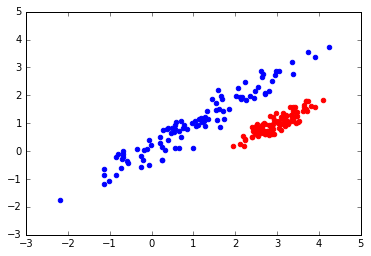In :
## OPTIMIZATION- in CvxPy!

a = Variable(d)
b = Variable()
t = Variable()

obj = Maximize(t)

x_constraints = [a.T * x[i] - b >= t  for i in range(m)]
y_constraints = [a.T * y[i] - b <= -t for i in range(n)]

constraints = x_constraints +  y_constraints + [norm(a,2) <= 1]

prob = Problem(obj, constraints)

prob.solve()
print("Problem Status: %s"%prob.status)

Problem Status: optimal

In :
## Define a helper function for plotting the results, the decision plane, and the supporting planes

def plotClusters(x,y,a,b,t):
# Takes in a set of datapoints x and y for two clusters,
#  the hyperplane separating them in the form a'x -b = 0,
#  and a slab half-width t
d1_min = np.min([x[:,0],y[:,0]])
d1_max = np.max([x[:,0],y[:,0]])
# Line form: (-a * x - b ) / a
d2_atD1min = (-a*d1_min + b ) / a
d2_atD1max = (-a*d1_max + b ) / a

sup_up_atD1min = (-a*d1_min + b + t ) / a
sup_up_atD1max = (-a*d1_max + b + t ) / a
sup_dn_atD1min = (-a*d1_min + b - t ) / a
sup_dn_atD1max = (-a*d1_max + b - t ) / a

# Plot the clusters!
plt.scatter(x[:,0],x[:,1],color='blue')
plt.scatter(y[:,0],y[:,1],color='red')
plt.plot([d1_min,d1_max],[d2_atD1min[0,0],d2_atD1max[0,0]],color='black')
plt.plot([d1_min,d1_max],[sup_up_atD1min[0,0],sup_up_atD1max[0,0]],'--',color='gray')
plt.plot([d1_min,d1_max],[sup_dn_atD1min[0,0],sup_dn_atD1max[0,0]],'--',color='gray')
plt.ylim([np.floor(np.min([x[:,1],y[:,1]])),np.ceil(np.max([x[:,1],y[:,1]]))])

In :
# Typecast and plot these initial results

if type(a) == cvxpy.expressions.variables.variable.Variable: # These haven't yet been typecast
a = a.value
b = b.value
t = t.value

plotClusters(x,y,a,b,t)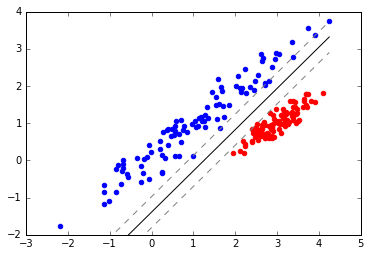We can see that the upper cluster had a large number of datapoints which were clipped, and thus became points on the support vector!

## Support Vector Classifiers¶

The discriminator works well for the case above, but doesn’t work when the clusters can’t be strictly separated. In real cases, there will be some crossover in the data between the classes. To handle this, we need to introduce variables which create slack in the constraints associated with points which fall on the ‘wrong’ side of our decision hyperplane.

Initially, we’ll still be trying to minimize the number of points which are inside of our ‘slab’ defined by $t$. This minimizes the number of support vectors, but makes the system more prone to variance as small movements in the support vectors can affect how our classifier is structured.

To address this, we’ll allow for a trade-off between having a minimizing the number of misclassified points (low bias) and maximizing the width of our slab (low variance).

### Initial formulation¶

We introduce vectors $u$ and $v$ which take the place of $t$, and create slack in the constraints for classifying the constraints. We want these to be sparse, and have positive entries:

\begin{align} \underset{a,b,t}{\text{minimize}} \quad & \mathbf{1}^T u + \mathbf{1}^T v \\ \text{subject to}\quad & a^T x_i – b \geq 1 – u_i, \; \forall i = 1,\dots,M\\ & a^T y_i – b \leq -1 + v_i, \; \forall i=1,\dots,N\\ & u \succeq 0, \; v \succeq 0 \end{align}

In :
## Generate data- this time, it can overlap!
# Relies on the centerpoints defined above
np.random.seed(2) #2 works well

orientation_x = np.random.rand(2,2)
orientation_y = np.random.rand(2,2)

x = x_center + np.dot(np.random.randn(m,d),orientation_x)
y = y_center + np.dot(np.random.randn(n,d),orientation_y)

# Check out our clusters!
ax = plt.subplot(111)
plt.scatter(x[:,0],x[:,1],color='blue')
plt.scatter(y[:,0],y[:,1],color='red')
ax.set_ylim([np.floor(np.min([x[:,1],y[:,1]])),np.ceil(np.max([x[:,1],y[:,1]]))])

Out:
(-1.0, 3.0)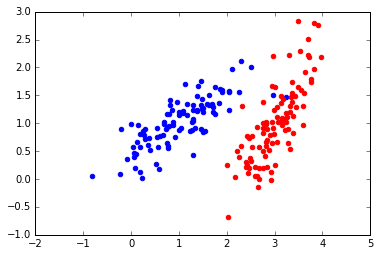In :
## OPTIMIZATION- in CvxPy!

a = Variable(d)
b = Variable()
u = Variable(m)
v = Variable(n)

obj = Minimize(np.ones(m)*u + np.ones(n)*v)

x_constraints = [a.T * x[i] - b >= 1 - u[i]  for i in range(m)]
y_constraints = [a.T * y[i] - b <= -1 + v[i] for i in range(n)]
u_constraints = [u[i] >= 0  for i in range(m)]
v_constraints = [v[i] >= 0  for i in range(n)]

constraints = x_constraints +  y_constraints + u_constraints + v_constraints

prob = Problem(obj, constraints)

prob.solve()
print("Problem Status: %s"%prob.status)
plotClusters(x,y,a.value,b.value,1)

Problem Status: optimal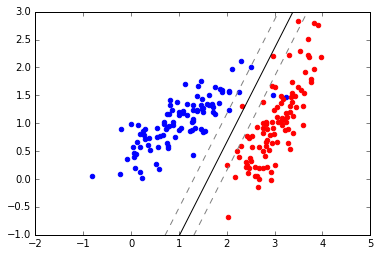We see that the decision plane makes intuitive sense- things seem to be working! Also, note that we’re trading off the penalties for all the points which are on the ‘wrong side’ of the slab- this means that the decision plane rests a bit closer to the blue cluster, as the red cluser is much more dense if it were to move over, and the weight would rapidly increase as more constraints are violated.

## Canonical Support Vector¶

This system is prone to variance, as the support vectors change slightly. Increasing the slab width would create a more robust classifier (reducing variance) at the expense of more bias (initial misclassification). We can do this by trading off between the magnitude of $a$ and the penalty for misclassification:

\begin{align} \underset{a,b,t}{\text{minimize}} \quad & ||a||_2 + \gamma \left( \mathbf{1}^T u + \mathbf{1}^T v \right) \\ \text{subject to}\quad & a^T x_i – b \geq 1 – u_i, \; \forall i = 1,\dots,M\\ & a^T y_i – b \leq -1 + v_i, \; \forall i=1,\dots,N\\ & u \succeq 0, \; v \succeq 0 \end{align}

In :
## Optimization- just a few modifications to our previous problem!

gamma = Parameter()
gamma.value = 0.4

obj = Minimize(norm(a,2) + gamma*(np.ones(m)*u + np.ones(n)*v) )
constraints = x_constraints +  y_constraints + u_constraints + v_constraints

prob = Problem(obj, constraints)

prob.solve()
print("Problem Status: %s"%prob.status)
## Plotting the results
plotClusters(x,y,a.value,b.value,1)

Problem Status: optimal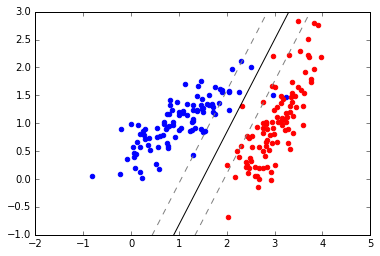In :
# We can change the value of Gamma around a bit
gamma.value = 0.05
prob.solve()
plotClusters(x,y,a.value,b.value,1)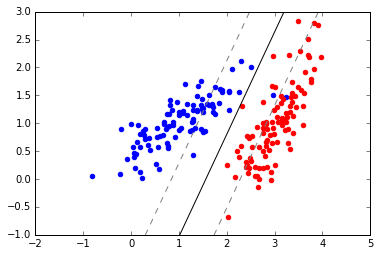# The Scikit-learn method¶

Let’s be realistic- my code isn’t optimized, I’m writing this in Python rather than C++, and relying on Cvxpy for optimization probably isn’t the fastest or most scalable. If you want an off-the-shelf package for deployment, head over to the Scikit-learn module for support vector machines. This is what the pros will be doing for prototyping, anyway.

In :
data   = np.vstack([x,y])
labels = np.vstack([ np.zeros([m,1]), np.ones([n,1]) ]).ravel()

In :
from sklearn import svm

clf = svm.SVC(kernel='linear', C=1-0.05)
clf.fit(data,labels)

Out:
SVC(C=0.95, cache_size=200, class_weight=None, coef0=0.0,
decision_function_shape=None, degree=3, gamma='auto', kernel='linear',
max_iter=-1, probability=False, random_state=None, shrinking=True,
tol=0.001, verbose=False)
In :
a1 = -np.matrix(clf.coef_).T
b1 = clf.intercept_
plotClusters(x,y,a1,b1,1)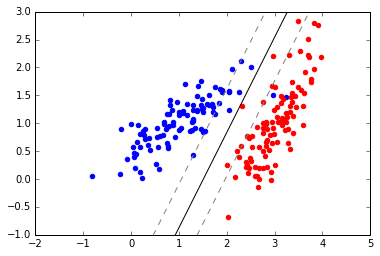# Conclusions¶

We just saw a hint of the potential of support vector machines to handle more complicated classification problems through use of alternative basis functions, known as the ‘kernel trick‘ – we’ll explore this in a future tutorial. Even with linear selection space, support vector machines are a great tool that scale well, take advantage of advances in convex optimization, and handle a wide variety of problems. Because we only need to preserve the points which define the dividing hyperplane, we can train on a very large dataset and then throw away all but the support vectors- this makes them extremely scalable.

However, they have limitations. They are binary classifiers, so managing more classes requires recursively separating classes. They require a good number of samples relative to the number of features- otherwise our hyperplanes will be underdefined.

If you’re interested in exploring more, I’d highly recommend checking out Stephen Boyd’s book, or seeing this Pythonprogramming.net tutorial which builds a SVM fully from scratch, including the creation of a gradient descent optimizer.